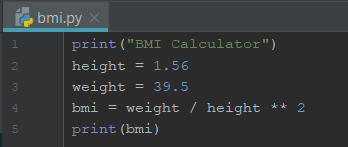# Day 7: Python – Calculate BMI

Today is a good time to make sure of numbers, arithmetic operation and exponentiation together to do a small tool to calculate BMI. Often, we can see this in any programming language exercise.

The requirements for the BMI calculation:

• height value
• weight value
• BMI formula (BMI = weight / height²)

Firstly, I use the 2 variables, height and weight to hold the both values.
Secondly, I apply the values into the BMI formula using arithmetic operators I learned previously.
Thirdly, I assign the outcome into a new variable called bmi.
Lastly, I print BMI.

Still remember, exponentiation? This is the right time to use it.

I used the IntelliJ to create a python file to run the BMI calculation and this is how I coded. With default height and weight values I stored in two variables, the bmi variable holds the arithmetic operation’s result and lastly, print() statement displays the result.Then, I hit the Run button to get my output. This combined what I have been shared in my first 7 days in learning Python. Below is the result:

BMI Calculator
16.231097961867192

Another example of calculating BMI using the Python numpy package as below, assuming we already have data in the collections, height_in (height in inches) and weight_lb (weight in lbs).

numpy package will be shared in my blog later. numpy package is one of the fundamental package for scientific computing with Python. It is often being used to carry out mathematical operation to entire collections and allows us to perform the computation fast.

We need to import the numpy package before we begin coding. See below:

```# height and weight are available as regular lists

# Import numpy
import numpy as np

# Create array from height_in with metric units: np_height_m
np_height_m = np.array(height_in) * 0.0254

# Create array from weight_lb with metric units: np_weight_kg
np_weight_kg = np.array(np_height_m) * 0.453592

# Calculate the BMI: bmi
bmi = np_weight_kg / np_height_m ** 2

# Print out bmi
print(bmi)
```

We also used the array in the numpy as you can see the code “np.array”. It converted the collections into an array before performed the arithmetic operation.

After that, in the same exercise, it prints out the BMIs of all players who BMI is below 21. Refer to below code:

```# height and weight are available as a regular lists

# Import numpy
import numpy as np

# Calculate the BMI: bmi
np_height_m = np.array(height_in) * 0.0254
np_weight_kg = np.array(weight_lb) * 0.453592
bmi = np_weight_kg / np_height_m ** 2

# Create the light array
light = np.array(bmi < 21)

# Print out light
print(light)

# Print out BMIs of all baseball players whose BMI is below 21
light = bmi < 21
print(bmi[light])
```

At line, light = np.array(bmi < 21)
it returns True and False values because of the condition checking. You can verify the output using the print(light).

Next, it print out the BMIs of the players who BMI is below 21. With the same condition checking again, we use print(bmi[light]) to list the BMI values below 21.

I realized I can make improvement on this code. Example:

• Input value instead of pre-assigned values.
• Add “My BMI is” text in front of the BMI value.
• Add filter to display the BMI value is ideal, underweight or overweight.

Summary of the day:

• Putting all I learned in the 7 days together.
• Introduction of numpy package.
• Introduction of numpy array and how to access its values.